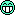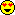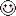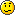# A (hopefully) practical approach to zero-delay-feedback filters

DSP, Plug-in and Host development discussion.
KVRist
239 posts since 22 Jan, 2007 from Germany
Good evening fellow KVR'ers. After reading through some posts here and looking through some Java code and text files of mine I considered to do another post on 'zero-delay feedback filters'.

This post is meant to give all people interested in the very useful 'delay-removal' for feedback paths a starting point without going into too much detail. It also is meant to be a reference for myself, so I know a fixed location to look for this stuff.

I won't go into the details of the bilinear integrator here, so it's left as an exercise to the reader to do a bilinear transform of H(s) = 1/s and bring it into DFII transposed form.

Most of the final formulas given here are more or less legal (C/C++/Java) code.

Anyways, it's a long post, a little bit messy but maybe it's helpful for others (and not only for me, as I'm using the described structures as my _only_ basic filter types).

For those who want to see a working implementation: Have a look here. This is the 'audio' package of my Java utils package.

Here we go (warning, long post ahead):

Bilinear integrator:

Code: Select all

``````    out = f * in + buf
buf = f * in + out

f   = tan(PI * fc / fs)
``````
Transfer function: H(s) = 1/s

Lowpass filter:

A lowpass filter can be constructed from an integrator in a closed feedback loop:

Code: Select all

``````    out             = integ(in - out)
out             = f * (in - out) + buf
``````

Now we eliminate the (normally implemented) unit delay in the feedback path by solving for 'out':

Code: Select all

``````    out             = f * in - f * out + buf
out * (1 + f)   = f * in + buf
out             = (f * in + buf) / (1 + f)
``````
As '1/(1+f)' is widely used here we define:

Code: Select all

``````    f2              = 1 / (1 + f)
``````
so our output function looks like this:

Code: Select all

``````    out             = (f * in + buf) * f2
``````
and the final filter:

Code: Select all

``````    out             = (f * in + buf) * f2
buf             =  f * (in - out) + out
``````
Transfer function: H(s) = 1 / (s + 1)

Derivation of the transfer function by using the closed loop transfer function:

Code: Select all

``````    Y(s)       H(s)
---- = -------------
X(s)   1 + H(s)*G(s)
``````

I switched 'G(s)' and 'H(s)' here to make clear which function we will substitue. 'G(s)' is the transfer function of the feedback path which is in our case:

Code: Select all

``````    G(s) = 1
``````

so we get:

Code: Select all

``````    Y(s)       1/s          1      1         1        1
---- = ------------- =  - * ------- = ------- = -----
X(s)   1 + 1/s*1        s   1 + 1/s   s + s/s   s + 1
``````

Highpass filter:

A high pass filter can be constructed by subtracting the lowpass output from the input:

Code: Select all

``````    out = in - lpf(in)
out = in - (f * in + buf) * f2
out = in - f * f2 * in - f2 * buf
out = (1 - f * f2) * in - f2 * buf
``````
we define:

Code: Select all

``````    f3  = (1 - f * f2)
``````

so our output function looks like this:

Code: Select all

``````    out = f3 * in - f2 * buf
``````

and the final filter:

Code: Select all

``````    out = (f *  in        + buf) * f2
buf =  f * (in - out) + out
out = in - out
``````
Transfer function: H(s) = s / (s + 1)

Derivation of transfer function:

Code: Select all

``````             1     (s + 1) - 1     s
H(s) = 1 - ----- = ----------- = -----
s + 1      s + 1      s + 1
``````

Lowpass filter with variable feedback:

For modelling of complex filters it can be useful to have a generic 1-pole lowpass filter with variable feedback. The transfer function of such a filter will look like this:

Code: Select all

``````    H(s) = 1 / (s + fb)
``````

where 'fb' is our feedback factor.

I'll just post the resulting formulas here, as the derivation is similar to that of the lowpass filter above:

Code: Select all

``````    f2 = 1 / (1 + f * fb)
``````

Output function:

Code: Select all

``````    out             = (f * in + buf) * f2
``````
Final filter:

Code: Select all

``````    out             = (f *  in             + buf) * f2
buf             =  f * (in - fb * out) + out
``````

Lowpass filter for resonant filter structures:

If we want to build a generic 2-pole ladder filter with feedback like this here:

Code: Select all

``````    in -+-> [ LPF ] -> [ LPF ] -+-> out
|                       |
+--------<-[*k]-<-------+
``````
we will also have to remove the unit delay in this feedback path by solving for 'out' in the following (lpf) equation:

Code: Select all

``````    out = lpf(in - k * out)
out = (f * (in - k * out) + buf) * f2
out = (f * in - f * k * out + buf) * f2
out = f * f2 * in - f * f2 * k * out + f2 * buf
out * (1 + f * f2 * k) = f * f2 * in + f2 * buf
out * (1 + f * f2 * k) = (f * in + buf) * f2
out = (f * in + buf) * f2 * (1 / (1 + f * f2 * k))
``````
The nice thing with this formula is that we can easily chain it to form ladder filters, I'll come back to this later.

Highpass filter for resonant filter structures:

Here's the same thing for the highpass filter:

Code: Select all

``````    out = hp(in - k * out)
out =  f3 * (in - k * out) - f2 * buf
out =  f3 * in - f3 *k * out - f2 * buf
out = (f3 * in - f2 * buf) * (1 / (1 + f3 * k))

``````

Filter chaining with feedback:

First, let's set up some stuff. The reason why I always said 'output function' and 'final filter' will be made clear here. Say we define a lowpass filter having three methods:

Code: Select all

``````    double output(double in)
This one returns the value of the 'output function'
without altering the state variables (buf).

double tick(double int)
This one updates the state variable and returns 'out'
('final filter').

double coef(double prev)
This one is used for filter chaining and will be explained
in detail just _now_.
``````

Let's have a look again at the feedback function for the LPF and HPF:

Code: Select all

``````    LPF: out = (f * in + buf) * f2 * (1 / (1 + f * f2 * k))
out = LPF(in) * (1 / (1 + f * f2 * k))
HPF: out = (f3 * in - f2 * buf) * (1 / (1 + f3 * k))
out = HPF(in) * (1 / (1 + f3 * k))
``````
It can be seen (left as an exercise for the reader) that we can chain multiple LPF/HPF using the coef function:

Code: Select all

``````    LPF: double coef(double prev) -> return prev * f * f2;
HPF: double coef(double prev) -> return prev * f3;
``````
Now, having all our methods ready, here's an example which chains two LPFs and two HPFs to build a resonant bandpass filter:

Code: Select all

``````    lpf0 and lpf1 are our LPFs
hpf0 and hpf1 are our HPFs
k is the feedback factor
``````

First we calculate our feedback coefficient (which is a simple chaining of 'coef' calls, as I mentioned before):

Code: Select all

``````    double fbcoef = 1.0 / (1.0 + k *
hfp1.coef(hpf0.coef(lpf1.coef(lpf0.coef(1)))));
``````

And our filter would now look like this:

Code: Select all

``````    double fb = fbcoef *
hpf1.output(hpf0.output(lpf1.output(lpf0.output(in))));
return hp1.tick(hpf0.tick(lpf1.tick(lpf0.tick(in - k * fb))));
``````
... and that's all. This chaining works for all combinations of LPFs and HPFs and even for different cutoff settings per pole.

If you chain the 'variable feedback LPF' you can even specify the pole positions for the filter, e.g.

Code: Select all

``````                       1
H(s) = ---------------------------
(s + 2) * (s + 1) * (s - 1)
``````

can be realized by chaining three 'vf LPFs' with 'fb' set to 2, 1 and -1 respectively.

Zero-delay SVFs as second building blocks:

To create more sophisticated filters we need at least two generic types of building blocks:

Code: Select all

``````    a generic one pole with variable feedback
a generic two pole (SVF) with variable coefficients
``````

Chaining these blocks with some different gain factors for each block or SVF output allows us to model (nearly) every transfer by just transfering the factors in the analogue transfer function into our coefficients.

Here's a generic SVF drawn using my amazing ASCII art skillz:

Code: Select all

``````         high             band
|                |
in ->-+-->-[ INTEG ]->-+->-[ INTEG ]-+-> low
|                |             |
[ *-1 ]--<-[ *k ]-<-+             |
|                              |
[ *-1 ]-----------<---------------+
``````

Here are the three transfer functions for the three outputs:

Code: Select all

``````                     1
Hlow(s)  = ---------------
s^2 + k * s + 1

s
Hband(s) = ---------------
s^2 + k * s + 1

s^2
Hhigh(s) = ---------------
s^2 + k * s + 1
``````

If now we define three gain parameters (Ghigh, Gband and Glow) and add all three SVF outputs multiplied by it's corresponding gain variable, we get the following transfer function:

Code: Select all

``````           Ghigh * s^2 + Gband * s + Glow
H(s) = ------------------------------
s^2 + k * s + 1
``````
I'm a bit lazy here and just post the final formulas for the zero-delay SVF (using bilinear integrators), but the derivation is basically the same as usual (solve for 'out'):

We define:

Code: Select all

``````    f   = tan(PI * fc / fs)
t   = 1 / (1 + k * f)
u   = 1 / (1 + t * f * f)
tf  = t * f
bl  -> second integrator buffer
bh  -> first integrator buffer
``````

now we get (final filter):

Code: Select all

``````    low     = (bl + tf * (bb + f * in)) * u
band    = (bb + f * (in - low)) * t
high    = in - low - k * band

bb      = band + f * high
bl      = low  + f * band
``````
I did not yet bother about deriving a feedback formula for the SVF to chain two or more in a feedback loop, but it won't make much sense here because the feedback is directly controlled by 'k'.

If you want more fancy filter structures, try to extend the SVF above to form a 3-pole or even 4-pole configuration. Linkwitz-Riley crossover is the recommended form for those (the 2-pole SVF above is in fact also a Linkwitz-Riley crossover).

The resulting transfer function for a 4-pole SVF with variable feedbacks, summed and multiplied by per-output gains looks something like this:

Code: Select all

``````           b0*s^4 + b1*s^3 + b2*s^2 + b3*s + b4
H(s) = ------------------------------------
s^4 + a1*s^3 + a2*s^2 + a3*s + a4
``````

Using the above filter you can (more or less) emulate every analogue filter by just filling in the analogue transfer function's coefficients into the 4-pole SVF without any fancy/nasty stuff and without the usual instabilities which would be caused by using 4-pole biquad sections instead.

Feel free to ask questions, also my response times might be long, so beware.

Edit: Fixed 1-pole filter 'buf' update code.
Last edited by neotec on Fri Aug 30, 2013 2:49 pm, edited 3 times in total.
... when time becomes a loop ...
---
Intel i7 3770k @3.5GHz, 16GB RAM, Windows 7 / Ubuntu 16.04, Cubase Artist, Reaktor 6, Superior Drummer 3, M-Audio Audiophile 2496, Akai MPK-249, Roland TD-11KV+

KVRAF
6463 posts since 12 Feb, 2006 from Helsinki, Finland
Preferred pronouns would be "it/it" because according to this country, I'm a piece of human trash.

KVRian
1085 posts since 8 Feb, 2012 from South - Africa
Personally - I say - the more the merrier! Thanks for the info neotec! There are hundreds of papers and blogs on Biquads - and a had to read alot of them just to 'get it' - so more on zdf is always good - even though it might be a dated subject to those that 'got it' - right off the bat. Still a bit boggeled sometimes about where and how to add non-linearities into the system of equations. Also - just for interist's sake - has anybody done a 'leapfrog' with trapezoidal integration?

KVRist

Topic Starter

239 posts since 22 Jan, 2007 from Germany
If you mean "The Art of VA Filter Design" then, yep, I know it, but seriously ... how many people do you think will understand this fully? And how many people will just run away after seeing the first pages full of mathematical formulas? Not everybody is a native methematics speakerApparently I seem to have missed the 'discussion', a link would be nice if you would be so kind.

I for myself understand stuff like that better when I see it in pseudo code or some algorithmic representation. Mathematical formulas are always harder to read/understand (at least for me) and a lot harder to transform into 'code' if you don't have a good starting point or fundamental DSP knowledge.

While I think that better understanding of DSP will give better results no one should be scared off by too much math. If one is interested in the topic, he mostly wants to see (hear) results quite fast ... the deeper knowledge comes over time.

So, sorry if I'm bothering the ones already knowing this stuff in and out but I bet there are a few guys who feel like me and will appreciate my (long) post.
Ichad.c wrote:Personally - I say - the more the merrier! Thanks for the info neotec! There are hundreds of papers and blogs on Biquads - and a had to read alot of them just to 'get it' - so more on zdf is always good - even though it might be a dated subject to those that 'got it' - right off the bat. Still a bit boggeled sometimes about where and how to add non-linearities into the system of equations. Also - just for interist's sake - has anybody done a 'leapfrog' with trapezoidal integration?
I'm quite happy with just having soft saturation on the state variables ('buf'). Sure, it does not sound the same as when they're in the direct output path, but the filter stays predictable and with some pre- and postprocessing you can even turn those filters into beasts. My priority is stability, predictability and performance.
Last edited by neotec on Tue Dec 18, 2012 12:22 pm, edited 1 time in total.
... when time becomes a loop ...
---
Intel i7 3770k @3.5GHz, 16GB RAM, Windows 7 / Ubuntu 16.04, Cubase Artist, Reaktor 6, Superior Drummer 3, M-Audio Audiophile 2496, Akai MPK-249, Roland TD-11KV+

KVRAF
2538 posts since 4 Sep, 2006 from 127.0.0.1
neotec: exactly, math is definately not a friend of mine, and i have a better chance of understanding pseudo-code and explanation
i've understood a lot from your previous posts on the subject of zero-delay first order LPFs, for which i gotta thank you again!

i respect mystran and the others, but my brain simply cannot cope with the stuff they juggle around

more and more i dig out some old KVR threads and re-read them for the Nth time and sometimes i understand another bit of what someone has said, which is a great thing for meIt doesn't matter how it sounds..
..as long as it has BASS and it's LOUD!

irc.libera.chat >>> #kvr

KVRist

Topic Starter

239 posts since 22 Jan, 2007 from Germany
Thanks anttoJust to make thinks clear: I don't mean to offend anyone and VAFilterDesign is also a valuable resource for myself, but that's not been always the case.

I started doing DSP about 10 years ago as a hobby project (and it still is a hobby, as I'm doing totally different things on my job). It took me years to understand most of the stuff. I was always looking for resources that explain all those heavy math stuff in simple terms and I still remember how frustrated I've become after failing to learn/understand the stuff I desperately needed.

I don't want anybody to believe that DSP is some kind of black magic. There were so many moments where I though: "hey, that's pretty easy once you understand it" but I had to learn it the hard way. You don't need to understand all the math that's going on to understand DSP ... and you can do pretty awesome things too once you realize that it's not as complicated as it looks.

In my opinion it's never wrong to share your knowledge in easily understandable terms to give newbies (which all of us have been sometime) the chance to grasp some basic knowledge, loose the fear of DSP and start to dig deeper.... when time becomes a loop ...
---
Intel i7 3770k @3.5GHz, 16GB RAM, Windows 7 / Ubuntu 16.04, Cubase Artist, Reaktor 6, Superior Drummer 3, M-Audio Audiophile 2496, Akai MPK-249, Roland TD-11KV+

KVRian
1085 posts since 8 Feb, 2012 from South - Africa
In "other thread's defense" - I was very thankful for mystran's nearly step-by-step mini-tutorial that used Maxima to illustrate how to solve the equations, it helped bucketloads - as Maxima is the one math proggie that is intuitive for me. As for "the book" - I get lost when it switches to complex numbersKVRist

Topic Starter

239 posts since 22 Jan, 2007 from Germany
Ichad.c wrote:In "other thread's defense" - I was very thankful for mystran's nearly step-by-step mini-tutorial that used Maxima to illustrate how to solve the equations, it helped bucketloads - as Maxima is the one math proggie that is intuitive for me.
That one sounds interesting (I'm doing all of the equation stuff by hand). Will have a search on the forum to see if I can find the posts you mentioned.
... when time becomes a loop ...
---
Intel i7 3770k @3.5GHz, 16GB RAM, Windows 7 / Ubuntu 16.04, Cubase Artist, Reaktor 6, Superior Drummer 3, M-Audio Audiophile 2496, Akai MPK-249, Roland TD-11KV+

KVRian
1085 posts since 8 Feb, 2012 from South - Africa
No need to search:

http://www.kvraudio.com/forum/viewtopic ... highlight=

A good walk-though is on page2. And BTW - by HANDKVRist

Topic Starter

239 posts since 22 Jan, 2007 from Germany
Thanks, I'm currently having a look at Maxima ... feeling a bit ashamed that it took me so long to discover this program(thanks mystran)

Already found out that my highpass equations can be simplified much further ...... when time becomes a loop ...
---
Intel i7 3770k @3.5GHz, 16GB RAM, Windows 7 / Ubuntu 16.04, Cubase Artist, Reaktor 6, Superior Drummer 3, M-Audio Audiophile 2496, Akai MPK-249, Roland TD-11KV+

KVRAF
7096 posts since 17 Feb, 2005
Good job! I will be testing this out soon.

KVRAF
6463 posts since 12 Feb, 2006 from Helsinki, Finland
neotec wrote:
If you mean "The Art of VA Filter Design" then, yep, I know it, but seriously ... how many people do you think will understand this fully? And how many people will just run away after seeing the first pages full of mathematical formulas? Not everybody is a native methematics speakerWell, I wasn't criticizing, just checking.Preferred pronouns would be "it/it" because according to this country, I'm a piece of human trash.

KVRAF
7096 posts since 17 Feb, 2005
I seem to have a problem with the one-pole LP. I implemented this in SynthMaker

Code: Select all

``````streamin input;
streamout output;
streamin cutoff;

float in, out;
float pi;
float fs;
float fc;
float f;
float f2;
float buf;

//initialize
stage (0) {
pi = 3.14159265359;
fs = 96000;
buf = 0;
}

in = input;
fc = cutoff;
f = tan1(pi * fc / fs);
f2 = 1 / (1 + f);
out = (f * in + buf) * f2;
buf = f * in + out;
output = out;
``````
If fc is set above about 6000 somewhere, then it goes unstable. fs is defined at 96000, and the DAW is running at 96000 also.

KVRist

Topic Starter

239 posts since 22 Jan, 2007 from Germany
Your code for updating 'buf' is bogus.

You're using the pure integrator formula, but you have to use the lowpass input, so:

Code: Select all

``buf = out + f * (in - out)``
A lowpass is an integrator with negative feedback: out = integrate(in - out), and the integrator is given by:

Code: Select all

``````out = f * in + buf
buf = f * in + out
``````
In the lowpass case this becomes

Code: Select all

``````out = f * (in - out) + buf
buf = f * (in - out) + out
``````
from which we solved to 'out' in the first equation.

This is the only problem I see right now.

Edit: I just saw that I wrote this down incorrectly in the starting post, I corrected the code for the final filter ... my fault... when time becomes a loop ...
---
Intel i7 3770k @3.5GHz, 16GB RAM, Windows 7 / Ubuntu 16.04, Cubase Artist, Reaktor 6, Superior Drummer 3, M-Audio Audiophile 2496, Akai MPK-249, Roland TD-11KV+

Mr Entertainment
12216 posts since 30 Apr, 2002 from i might peeramid
*edit* i defer to OP
you come and go, you come and go. amitabha neither a follower nor a leader be tagore "where roads are made i lose my way" where there is certainty, consideration is absent.